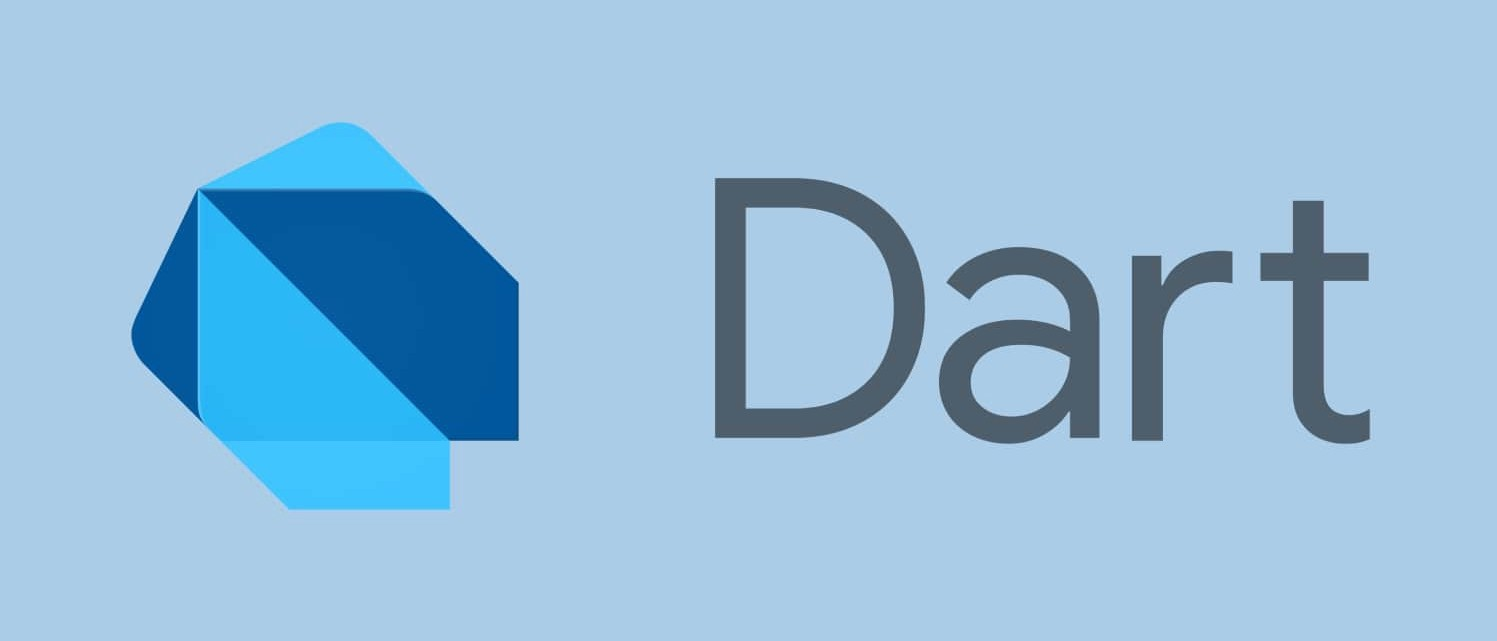### Numbers in Dart #

In the previous video, I told you there are two possible numbers data type is there.

• Integer (Integer Number)
• Double (Rational Number)

How to define/declare a variable ?main.dart
``````int variable_name_1;
double variable_name_2;
``````

Once you declare a variable with it’s type name after that computer will remember that this label is of type integer or double or whatever type you have wrote in the program.

In dart `integer` is refereed as `int`

#### Example 1 #

In this example we will define/declare a variable `a` of int(integer number) type, after that we will assign some value to `a`.main.dart
``````int main(){
int a;
a = 2;
print(a);
}
``````

#### Example 2 #

In this example we will define/declare a variable `x` of double(decimal number) type, after that we will assign some value to `x`.main.dart
``````int main(){
double x;
x = 3.14;
print(x);
}
``````

You can assign some value to the variable at the time of variable declaration also.

#### Example 3 #

Here I defined a variable `a` with the initial value of 2.main.dart
``````int main(){
int a = 2;
print(a);
}
``````

#### Example 4 #

Here I defined a variable `x` with the initial value of 3.14.main.dart

``````int main(){
double x = 3.14;
print(x);
}
``````